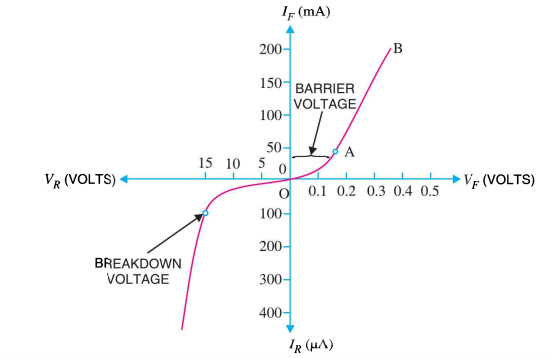# Kindly answer the question. (a) Draw V-l characteristics of a p-n Junction diode. (b) Differentiate between the threshold voltage and the breakdown voltage for a diode. (c) Write the property of a junction diode which makes it suitable for rectification of ac voltages.

Solution
a. V-I characteristics of p-n junction diodeV and Vare forward and reverse bias voltage respectively, and I and Iare forward and reverse bias current respectively.
b.
Threshold Voltage or barrier voltage is at which in forward bias condition the current through the PN junction diode increases rapidly. It is also termed as knee voltage or cut in voltage.
In a reverse biased condition, if the voltage is increased gradually through a diode, initially the current (in microamp range) is very low. If we increases the reverse potential then there comes a specific voltage when the diode suffers a breakdown, then the reverse current starts increasing significantly at this specific voltage, that's reverse potential is known as breakdown potential or breakdown voltage.
c.
The resistance of PN junction diode is not constant, it changes according to the applied potential difference and polarity.  To be specific the ideal diode has zero resistance when it is kept in forward biased and has infinity resistance when it is kept at reverse biased. So this helps the flow of current in one direction making it a suitable candidate to be a rectifier.

• 0
What are you looking for?